# Formula Forensics. No 007 – Sumproduct

Share

One of the most asked questions within the posts and Forums at Chandoo.org is “How Does Sumproduct work ?”.

Rahul recently asked for an example in Excels Sumproduct Formula post;  Comment No. 55.

So today in Formula Forensics we will take a look at just that with a few worked examples.

## Sumproduct

Excels help defines Sumproduct as: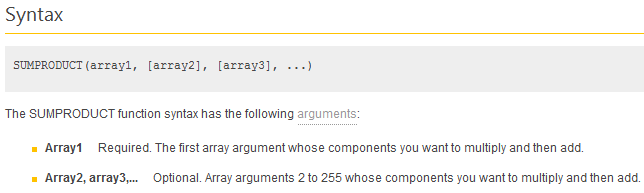So what are these arrays referring to:

An array in Excel can be :

A manual Array:     {10;20;30}

A Range:              A1:A3

A Named Range: MyRange1

Where MyRange1 is defined as a defined range in the Name Manager.

A Named Formula: MyRange2

Where MyRange2 is defined as a Formula returning a range in the Name Manager.

Lets look at each

You can follow along in the Example file on Sheet1

An Array

In C2 type: =SUMPRODUCT({10;20;30})

Excel will display 60, which is the Sum of the array elements =10+20+30

A Range

C7:          =Sumproduct(C4:C6)

Excel displays 60, which is the Sum of the cells from the range C4:C6 =10+20+30

A Named Range

In the Name Manager or Name Box define a Named Range

MyRange1:         =Sheet1!\$C\$4:\$C\$6

Then in C10 type:

C10: =Sumproduct(MyRange1)

Excel displays 60, which is the Sum of the range elements =10+20+30

A Named Formula

In the Name Manager define a Named Formula

MyRange2          =OFFSET(Sheet1!\$C\$3,1,0,3,1)

Then in C12 type:

C12:       =Sumproduct(MyRange2)

Excel displays 60, which is the Sum of the range elements from cells C4:C6 =10+20+30

You may be asking why use Sumproduct when we can use a simple Sum to add up 3 numbers?

The answer is to show you what Sumproduct is doing, it is Adding up each Array element.

### What about the “Product” part of Sumproduct ?

Remember back at the start where we saw the Definition of Sumproduct,

SUMPRODUCT(array1, [array2], [array3], …)

Only Array 1 is required, Array 2, Array 3 etc are optional, that’s what the square brackets [ ] mean.

## Multiple Arrays

Goto Sheet 2 in the Example file:

We will look at a simple example using two arrays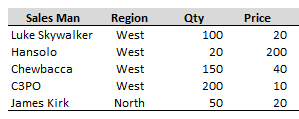The data consists of Sales data.

Often we want to know what the total sales are

We do this by  adding a Sales column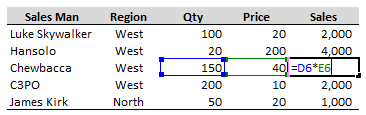Which multiplies the Qty and Price columns

And then Sum (Add) up this new column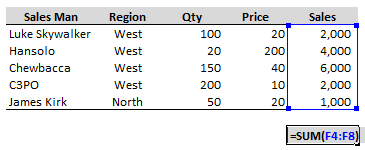Returning our Total Sales of 15,000

Now we can manually check the above as the numbers are simple eg: 100*20 = 2,000 etc

And we can sum up the Sales and see that we in fact had total sales of 15,000

Well this is exactly what Sumproduct is made to do:

In a Blank cell enter: =SUMPRODUCT(D4:D8,E4:E8)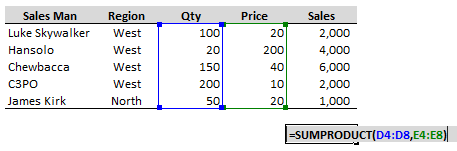Excel will return 15,000.

So what is Sumproduct doing?

Lets look inside and see what’s going on

In the Example File, Sheet2, H1 there is a copy of the data laid out as below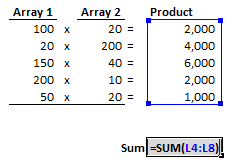Note that our formula =SUMPRODUCT(D4:D8,E4:E8)

Has two Arrays

Array 1: D4:D8

Array 2: E4:E8

Note that each corresponding Array Element is multiplied together

100 x 20

20 x 200 etc

These are the products of the two Arrays

Finally the Products are Added together and the correct answer 15,000 is returned.

So Sumproduct is the Sum of the Products of the Arrays

Of course we can extend that to a large number of Arrays, columns in this case, if we wish.

## Sumproduct with Logic

In the above two examples we saw that Sumproduct can Sum a single Array and can Sum the Product of two or more Arrays.

We can use that to our advantage and build logic into the arrays, allowing us to optionally include some array elements and leave out others.

### How?

Sumproduct will always add up the product of all Arrays.

So by including an Array where the elements within the Array that we don’t want to Sum are Zero and the Elements within the array that we do want to Sum are 1 we can control what is included in the final Summation.

Goto our Example File on Sheet3

Lets say we only want to include the Sales from our Northern Region

One way to do this is to purely delete the other entriesBut what if we could do that without altering our worksheet or there are thousands of rows of data?

This is where Sumproduct comes into its own.

What we need to do is add some logic to our equation, effectively doing:Lets try it with Sumproduct

In Cell F12: type =SUMPRODUCT(D4:D8,E4:E8,{FALSE;TRUE;FALSE;FALSE;TRUE})

Excel displays a –

Excel doesn’t know what to do with the True/False and so converts them to 0

We can force excel to evaluate these as numbers by adding a simple “1*”

In F14: Type =SUMPRODUCT(D4:D8,E4:E8,1*{FALSE;TRUE;FALSE;FALSE;TRUE})

Excel now displays 5,000 the total sales from the North

To see what has happened in F16 type: 1*{FALSE;TRUE;FALSE;FALSE;TRUE}, but don’t press Enter press F9 instead.

Excel displays ={0;1;0;0;1}

The use of the 1* has converted each of the Array elements from a True/False to a 1,0 respectively.

So our 3 arrays are now: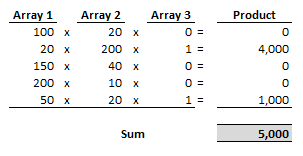Now adding an Array of 1*{FALSE;TRUE;FALSE;FALSE;TRUE} every time we wanted to add some numbers isn’t a practical solution.

Excel has the ability to work construct an Array on our behalf!

In E18: enter  =SUMPRODUCT(D4:D8,E4:E8,1*(C4:C8=”North”))

Excel will display 5,000

So 1*(C4:C8=”North”) is exactly equal to our previous array 1*{FALSE;TRUE;FALSE;FALSE;TRUE}

1*(C4:C8=”North”) = 1*{FALSE;TRUE;FALSE;FALSE;TRUE}

At the heart of this is that Excel is evaluating each cell in the Range: C4:C8 against our required logic =”North” and setting up an Array for us internally.

### Simplify

The power of Sumproduct is therefore in that we can now simplify and extend

In cell E20 type: North

In cell F20 type: =SUMPRODUCT(D4:D8,E4:E8,1*(C4:C8=E20))

Excel will display 5,000

This simple addition allows us to vary the Summation based on the value in E20

We don’t need to multiply our logic array by 1, we can actually use any number or another Array.

In cell F22 type: =SUMPRODUCT(D4:D8,(E4:E8)*(C4:C8=E20))

This works as (C4:C8=E20) is returning an Array of True/False which get converted to an array of 1/0’s when subject to any maths.

The Math in this case is the multiplication by the 2nd Array (E4:E8)*(C4:C8=E20)

In Cell F24 type: =SUMPRODUCT(Qty, Price *(Region=SalesRegion))

Excel will display 5,000

But notice that by using Named Ranges/Formula how simple the logic of the equation has now become.

## Rahul’s Question (Multiple Criteria):

In Comment No. 55: Rahul asked, “Can you give an example work sheet of above example

Sheet 4 in the Example File is the answer.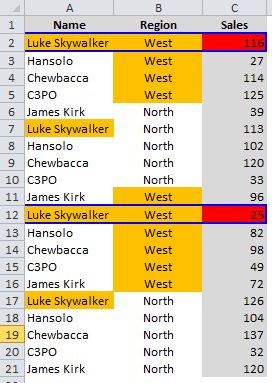In Cell C23: type: =SUMPRODUCT(- -(A2:A21=”Luke Skywalker”),- -(B2:B21=”West”),C2:C21)

Excel will display 141, which is the sum of the Sales made by Luke Skywalker in the West Region.

However using what was learned above, this is better simplified to:

C26: =SUMPRODUCT((Name=SalesMan)*(Region=SalesRegion)*Sales)

## The Double Unary

In the formula above Chandoo has used what is known as a Double Unary, which is 2 – signs next to each other (I have inserted a space above to make it more legible).

Two – signs are the same as saying

– -(A2:A21=”Luke Skywalker”) = -1 x -1 x (A2:A21=”Luke Skywalker”)

-1 x -1 is 1

Technically this is the most efficient way for Excel to perform any maths on the Array

– -(A2:A21=”Luke Skywalker”)

So that the Array of true/Falses made by (A2:A21=”Luke Skywalker”) is converted to an Array of 1/0’s for use in Sumproduct.

At the slight expense of speed but for improved readability and understandability by others I prefer the use of 1* instead of – – and you will mostly see that convention in my posts.

Chandoo:            – –(A2:A21=”Luke Skywalker”)

Hui:                       1*(A2:A21=”Luke Skywalker”)

In fact any maths performed on the array will convert its contents to an array of 1/0’s, so long as the maths doesn’t change the Arrays values

For a real good discussion on this topic have a look at the post The Venerable SUMPRODUCT at ExcelHero.com

http://chandoo.org/wp/2009/11/10/excel-sumproduct-formula/

http://chandoo.org/wp/tag/sumproduct/

## OTHER POSTS IN THIS SERIES

You can learn more about how to pull Excel Formulas apart and what makes them tick in the following post:

Formula Forensic Series:

## FORMULA FORENSICS NEEDS YOUR HELP !

I am running out of ideas for Formula Forensics and so I need your help.

If you have a neat formula that you would like to share and explain, try putting pen to paper and draft up a Post as Luke did in Formula Forensics 003. or like above.

If you have a formula that you would like explained but don’t want to write a post also send it in to Chandoo or Hui.

## XMAS BREAK

This will be the last Formula Forensics Post for 2011, but rest assured that we will be returning in early 2012.

I’d like to take the opportunity to thank Chandoo for allowing me the space and freedom to post pretty much what ever I’ve wanted at Chandoo.org. I hope you have enjoyed my contributions to the Chandoo.org community over the past year.

On behalf of Eva and myself I’d like to wish you all a very Merry Xmas and a Happy and Safe New Year ahead

### Get FREE Excel + Power BI Tips

Simple, fun and useful emails, once per week.

Learn & be awesome.

### Welcome to Chandoo.org

Thank you so much for visiting. My aim is to make you awesome in Excel & Power BI. I do this by sharing videos, tips, examples and downloads on this website. There are more than 1,000 pages with all things Excel, Power BI, Dashboards & VBA here. Go ahead and spend few minutes to be AWESOME.

Read my storyFREE Excel tips book

I will be presenting at two events in London in April 2020. Come & join me.

Chandoo is an awesome teacher
5/5

– Jason

Still on fence about Power BI? In this getting started guide, learn what is Power BI, how to get it and how to create your first report from scratch.

### 29 Responses to “Formula Forensics. No 007 – Sumproduct”

1.oldchippy says:

Very well explained Hui... you make a good teacher.

Merry Christmas and Happy New Year to one and all.

2.Bhushan Sabbani says:

Hi Chandoo.

Awesome article i have the doubt why we use the - sign
=SUMPRODUCT(- -(A2:A21= "Luke Skywalker"), - -(B2:B21="West"), C2:C21).

What is the use of the minus (-) sign if we remove the minus sign then the value come to 0.

3.Luke M says:

@Bhushan
The first minus symbol converts this:
(TRUE,FALSE,FALSE,TRUE)
into this:
(-1,0,0,-1)
But since we want positive values, the second minus sign is used to convert it to:
(1,0,0,1)

4.Luke M says:

Concerning the debate of using double unary versus multiplying the arrays, the ONLY situation I've run into where it makes a difference is if you're multiplying different sized arrays (ie, when you are using a row & column criteria check). Referring to the first example, let's say I wanted to know the sum of all the data for Qty column for the North region. This formula:
=SUMPRODUCT(- -(C4:C8="North"),- -(D3:E3="Qty"),D4:E8)

will error out. However, this formula:
=SUMPRODUCT((C4:C8="North")*(D3:E3="Qty")*D4:E8)
works just fine.

When I step through the calculation of both, they appear identical. Anyone able to cast some light onto the difference?

5.Luke M says:

Grr. There should be 3 double unary in the first formula. Comment got abbreviated automatically. =(

6.Hui... says:

Hi Luke
Re: Your problem with the Sumproducts - Comment #4
This gets into advanced Sumproduct area, but I will try and simplify it.
The correct formula =SUMPRODUCT((C4:C8=”North”)*(D3:E3=”Qty”)*D4:E8)
works as the 3 arrays are directly multiplied
Firstly =(C4:C8=”North”)
produces an array of {0;1;0;0;1}
Now the important part is that the semi colons ;'s represent Rows in the array
so the array {0;1;0;0;1} is 1 column x 5 rows
The next section (D3:E3=”Qty”)
returns {1,0}
Now the , represents new columns
so the array {1,0} is 2 columns of 1 row each
when these two (C4:C8=”North”)*(D3:E3=”Qty”) are multiplied
You get a product Array of {0,0;1,0;0,0;0,0;1,0}
Note the placement of the ,'s and ;'s
this is 2 columns of 5 rows
or
0, 0
1, 0
0, 0
0, 0
1, 0
.
Now when it is multiplied by the final area D4:E8
.
0, 0 x 100, 20
1, 0 x 20, 200
0, 0 x 150, 40
0, 0 x 200, 10
1, 0 x 50, 20
.
you only get matches for the Qty and North (In Bold)
.
Now the #Value! equation =SUMPRODUCT(--(C4:C8="North"),--(D3:E3="Qty"),D4:E8)
= --(C4:C8="North") = {0;1;0;0;1}
a 5 Row, 1 Column Array
= --(D3:E3="Qty") = {1,0}
a 1 Row, 2 Column Array
= (D4:E8) = {100,20;20;200,150;40,200;10,50;20}
a 5 Row, 2 Column Array
Because the 3 arrays are of different sizes Sumproduct spits out an error
.
The first equation =SUMPRODUCT((C4:C8=”North”)*(D3:E3=”Qty”)*D4:E8)
works as sumproduct is only processing 1 Array
The maths of the 3 blocks are all part of a single array and so the 3 ranges are processed as described above returning a 5 x 2 array which is then processed by sumproduct as expected
.
I hope the above helps in some small way.

7.Luke M says:

Hi Hui!
Thanks, that explanation was wonderful!

8.Mike says:

A very useful twist I have found is to be able to divide as well as multiply for example dividing a series of monthly totals (Col A) by the number of days in the month (Col B) to give daily averages. daily rate = (A1:A12,(B1:B12)^-1)

•Saurabh Aggarwal says:

Thank you Mike, this is a very cool twist.. respect..

9.Hui... says:

@Mike
Just be careful with divisions
as the Sumproduct(divisions) < > Sumproduct()/Count()

10.Tamoghna says:

Hi Hui,
I like your post very much and this post is no exception.
It will be very helpful if you discuss " MOD " function in detail.
I have seen both you and chandoo use this function a lot in a variety of cases.

11.Bruce says:

Sumproduct Is my favourite excel function. It's the poor mans array function.

Here's an interesting usage.

http://ramblings.mcpher.com/Home/excelquirks/quirky-functions/sumproduct/sumproduct-strangeness-1

Bruce

12.Modeste says:

Hi Hui ...
don't omit also the "venerable MVP Bob Phillips"
http://www.xldynamic.com/source/xld.SUMPRODUCT.html#refs

13.Les says:

Hui,
THANK YOU! While I LOVE this site for EVERY thing that it does... until today, I was still not using more than the most primitive 'Sumproduct' form...

YOur 'step by un-folding' step methodology made it easy for me to get to at least, ONE more step...:-)!

THX!

Les

14.Andrew says:

I've done similar things with array formulas:
{sum(qty_range*price_range*logic_range)} would get the same result.

It works very well with AND logic
logic1*logic2 is equivalent to AND(logic1,logic2)
e.g. luke AND West

I've never had much luck with OR logic.
e.g Luke OR Han
(Luke OR Han) AND West

Do you have any tips on making ORs work?

15.Andrew says:

I think i just figured OR out for myself.
Just an extension of the double unary

=TRUE + TRUE returns 2
=NOT(NOT(TRUE+TRUE)) returns TRUE
=--NOT(NOT(TRUE + TRUE)) returns 1

These last two could be used for counting or summing.

Or I could just use the OR() formula correctly to get the same result.

16.Hui... says:

@Andrew
Or is implemented using a +
so in the example here
=SUMPRODUCT((C4:C8="North")+(C4:C8="West"),F4:F8)
will retrieve the Sales where the Region = North or Region = West

17.Lee says:

@Andrew (post 15) and @Hui (post 16)

As will

=SUMPRODUCT((F4:F8)*(C4:C8={"North","West"}))

18.andy holaday says:

Hui, Great exposé! When I first saw that SUMPRODUCT could be used for conditional aggregates, I totally fell in love.
But, one application of SUMPRODUCT I seldom see mentioned is determining a weighted average. I think this hews closer to the original intent of the function -- not to detract from other creative uses, but I think everyone should understand this important application.
I probably can't do it justice in this comment post, but I will try:
Suppose in A2:A4 you have sales, and in B2:B4 you have tax rates, and you are interested in the average tax rate for the book of sales. You can't just average over column B. Why not? Easier to understand with example:
Sales ... Tax Rate
1000 ... 0.04
10000 ... 0.00
100000 ... 0.08
If we took a simple average of the tax rates we would get 0.04. But this is not the correct average tax incurred on our book. The overall tax incurred is a function of (weighted by) sales -- notice the last row (largest sales and highest tax rate) has a large influence on the overall tax rate!
To calculate the average tax rate "the long way" we could add helper column C = A * B (i.e., the taxes incurred):
... 40
... 0
... 8000
sum over C to get total tax: 8040
Dividing total tax (8040) by total sales (111000) we get 0.07243. This is close to 0.08, which is what we would expect given the high tax rate of 0.08 leveraged by the highest sales of 100000.
Now, notice what we did:
The total under helper column C could also be expressed as SUMPRODUCT(A2:A4,B2:B4), literally summing the pair-wise products of A and B.
Then, we divided by SUM(A2:A4) to get the average tax rate.
So, instead of figuring the weighted average tax rate the "long way" we could simply place in one cell: =SUMPRODUCT(A2:A4,B2:B4)/SUM(A2:A4).
Hope this helps.

19.Jimhemm says:

Someone please solve the mystery for me.....

I am looking for a financial formula with a little twist. Let say I want to Deposit a sum of money (\$500K) into an account that earns a guaranteed interest rate that veries(GIR increases every 5 years by .5% Starting with a 1.5%) This account will pay out a level amount yearly for a stated number of years starting immediately till the account = \$0 (Lets say 21 years). Question… What is the level amount?

A typical formula with a fixed GIR would be:
PMT(rate,nper,pv,[fv],type)
PMT(?,21,\$500,000,,1)
As you can see I am not sure how to add a rate that changes every 5 years. Can someone help me with this?

20. [...] know from Formula Forensics 007 that Sumproduct, Sums the Product of the Arrays, and that when there is only 1 array it simply sums [...]

21. [...] know from Formula Forensics 007 that Sumproduct, Sums the Product of the Arrays, and that when there is only 1 array it simply sums [...]

22.Catherine says:

Thank you for inviting us to submit a formula and an explanation or a formula that needs explaining.

I will submit the next formula that stumps me in the spreadsheets I inherited. 😀

You guys are the best!

Catherine

23. [...] Lets pull it apart and see what is inside. =SUMPRODUCT(SUBTOTAL(3, OFFSET(C7:C13,ROW(C7:C13)-MIN(ROW(C7:C13)),,1)), – -(C7:C13=B2)) The formula is based on the Excel Sumproduct() function which we examined in Formula Forensic 007. [...]

24. [...] we saw in Formula Forensics 007, Sumproduct, Sums the Products of the included [...]

25.senthil says:

Here is my problem definition & expecting the answer at COL10
(22.692 for the given example)
COL1 COL2 COL3 COL4 COL5 COL6 COL7 COL8 COL9 COL10
A A B A B C B C A 22.692

COL1 .333 A 10
COL2 .333 B 9
COL3 .333 C 8
COL4 .333 D 7
COL5 .333 I 0
COL6 .333
COL7 .156
COL8 .156
COL9 .156

I tried with SUMPRODUCT function but it's not working fine.

•Hui... says:

@Senthil

I think this is what your after:
```COL1 COL2 COL3 COL4 COL5 COL6 COL7 COL8 COL9 COL10 A A B A B C B C A 10 10 9 10 9 8 9 8 10 0.333 0.333 0.333 0.333 0.333 0.333 0.156 0.156 0.156 22.860 ```
But this gives me 22.860 not 22.692

can you confirm?

26.Abhijeet says:

Hi i am using this formula for Overlap the dates =SUMPRODUCT((G3=\$B\$3:\$B\$100)*(F3=\$A\$3:\$A\$100))

but i want which dates are overlap that data i want can any one help me

27.Ufoo says:

Hui thanks for this post. I need your assistance in the sumproduct portion in this formula: =INDEX(\$E\$5:\$E\$17,SUMPRODUCT(SMALL((\$C\$5:\$C\$17="Josh")*(ROW(\$C\$5:\$C\$17)-4),2+COUNTIF(\$C\$5:\$C\$17,"Josh")))). It was posted by Chandoo for finding the second sales of Josh without using the helper column. The excel sheet with the question and answer, courtesy of Chandoo.org: http://chandoo.org/img/f/vw/vlookup-2nd-value-instructor-copy.xls. Again thanks a lot.

### Get FREE Excel & Power-BI Newsletter

One email per week with Excel and Power BI goodness. Join 100,000+ others and get it free.